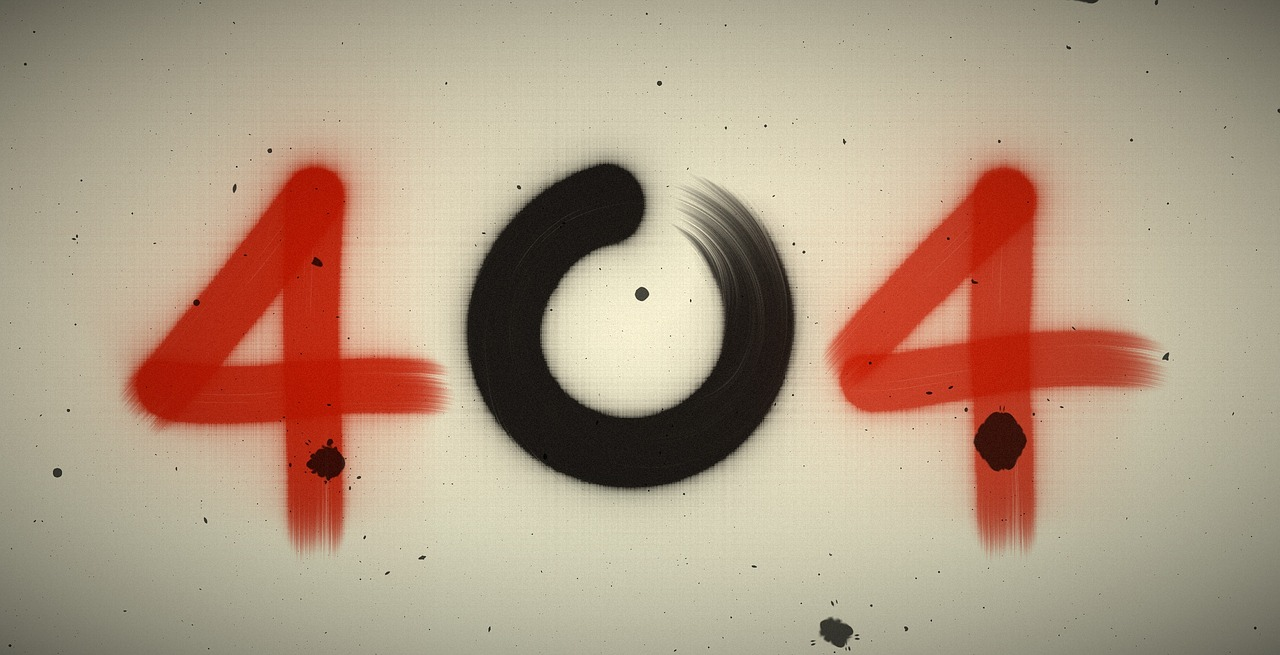## Post per Page

Black holes have a complex shape. Inside, gravity exists in three dimensions. But black holes are also connected to outside particles and magnetic fields that only exist in two dimensions. So how can a black hole exist in both two dimensions and three dimensions at the same time?

Scientists say there’s a mathematical phenomenon at work here, called the “holographic duality theory.” Juan Maldacena, an Argentine theoretical physicist, discovered the concept in 1997, which states that events inside a space with gravity (like a black hole) are mathematically equivalent to gravity-free events on the surface of that space that involve particles.

In other words, the holographic duality theory could hold the secret link between particle physics—the study of tiny particles that make up all matter—and Einstein’s theory of general relativity, which states that gravity arises from the curvature of space and time. In new research, published last month in the journal PRX Quantum, University of Michigan scientists look for support for this holographic duality theory inside of black holes. To understand, we have to review some pretty simple math and then apply the science.

Think back to basic calculus. There are lines, which are represented by x and y. Then there are curves, represented by x² and y². And if we raise those variables by one more power, we have three-dimensional surfaces and shapes. The relationship between dimensions is key to calculus, as is the relationship from the finite to the infinite.

How does that relate to black holes? Well, one expression of string theory (a theoretical framework in physics where point-like particles are replaced with one-dimensional objects called strings) and quantum theory aims to unify the two- and three-dimensional aspects of the real world as a way to help calculate and understand certain complex phenomena. The results could marry the standard model of particle physics and the theory of general relativity, bringing us full-circle: this is holographic duality.

The University of Michigan explains in a press release:

To envision this, think again of the black hole, which warps space-time because of its immense mass. The gravity of the black hole, which exists in three dimensions, connects mathematically to the particles dancing above it, in two dimensions. Therefore, a black hole exists in a three-dimensional space, but we see it as projected through particles. Some scientists theorize our entire universe is a holographic projection of particles, and this could lead to a consistent quantum theory of gravity.

In other words, ideas that don’t coordinate well when everything is three-dimensional may work better together when things like black holes are instead calculated in two dimensions. That can turn a three-dimensional black hole with no standard “particles” into a two-dimensional idea that can be measured by using particles.

“In Einstein’s General Relativity theory, there are no particles—there’s just space-time. And in the Standard Model of particle physics, there’s no gravity, there’s just particles,” University of Michigan physicist Enrico Rinaldi says in the statement. “Connecting the two different theories is a longstanding issue in physics—something people have been trying to do since the last century.”

String theory is one way scientists are seeking to unify the two disparate models of our universe. In string theory, particles like atoms are represented by one-dimensional “strings” that are, in turn, compiled into huge two-dimensional matrices. (A matrix, in science and math, is an n-by-n array of numbers or values.)

So putting the pieces all together, what we have is a two-dimensional grid of weighted values that describe the surface of the black hole that lies beneath. And from there, scientists can use mathematical ideas to explore the number grid the same way they would anything else. In this case, they used quantum operators, called qubits, and a running neural network to identify the least energy state of a given matrix model.

The scientists hope that the matrix models, which describe what one kind of black hole could look like inside, will help shed “light,” so to speak, on the event horizon of these special black holes—and beyond. “What is on the event horizon for a black hole? Where does it come from? Answering these questions would be a step towards realizing a quantum theory of gravity,” Rinaldi explains.